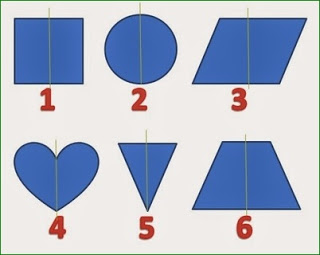Categories

# Odd One Out Shape Riddle

Which of the shape is the odd one out?Try to find out answer. There is some logic behind this. try to crack that logic and you will find the answer. If you still cannot solve it then scroll down to find the solution.

SOLUTION:

Third Image

Explanation
It’s the only image that is not symmetric around the vertical axis as illustrated in the picture below.Search Keywords:

Find odd one out puzzles

Odd one out riddles and answers

Find odd one out riddles with answers

Odd one out puzzles and answers

Odd one out puzzles with answers

Odd one out structure and answer riddles